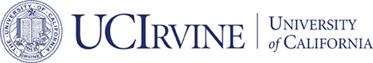# Accepted## Convergence Analysis of the Fast Subspace Descent Methods for Convex Optimization Problems

### Long Chen, Xiaozhe Hu, and Steven M. Wise

#### Mathematics of Computation

ABSTRACT:

```The full approximation storage (FAS) scheme is a widely used
multigrid method for nonlinear problems. In this paper, a new
framework to design and analyze FAS-like schemes for convex
optimization problems is developed. The new method, the Fast Subspace
Descent (FASD) scheme, which generalizes classical FAS, can be recast
as an inexact version of nonlinear multigrid methods based on space
decomposition and subspace correction. The local problem in each
subspace can be simplified to be linear and one gradient descent
iteration (with an appropriate step size) is enough to ensure a global
linear (geometric) convergence of FASD.
```